# Test if Value Exists in a Range in Excel & Google Sheets

This tutorial demonstrates how to use the COUNTIF function to determine if a value exists in a range.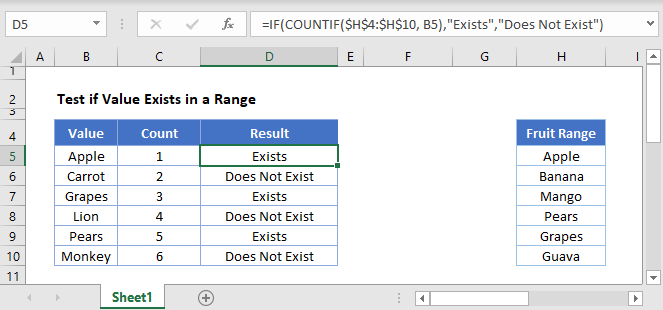## COUNTIF Value Exists in a Range

To test if a value exists in a range, we can use the COUNTIF Function:

``=COUNTIF(Range, Criteria)>0``

The COUNTIF function counts the number of times a condition is met. We can use the COUNTIF function to count the number of times a value appears in a range. If COUNTIF returns greater than 0, that means that value exists.

``=COUNTIF(\$E\$3:\$E\$9,B3)``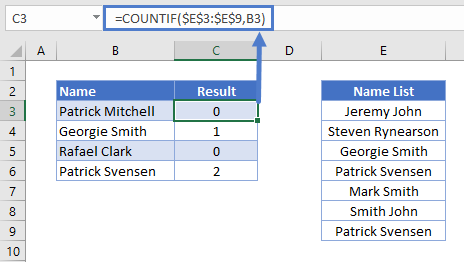By attaching “>0” to the end of the COUNTIF Function, we test if the function returns >0. If so, the formula returns TRUE (the value exists).

``=COUNTIF(\$E\$3:\$E\$9,B3)>0``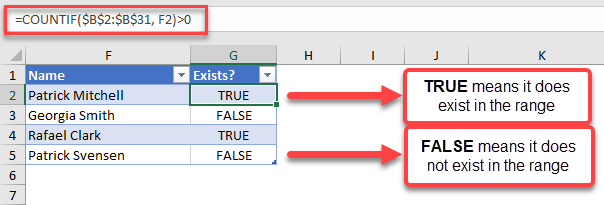You can see above that the formula results in a TRUE statement for the name “Patrick Mitchell. On the other hand, the name “Georgia Smith” and “Patrick Svensen” does not exist in the range \$B\$2:\$B\$31.

You can wrap this formula around an IF Function to output a specific result. For example, if we want to add a “Does not exist” text for names that are not in the list, we can use the following formula:

``=IF(COUNTIF(\$B\$2:\$B\$31, Name)>0, “Exists”, “Does Not Exist”)``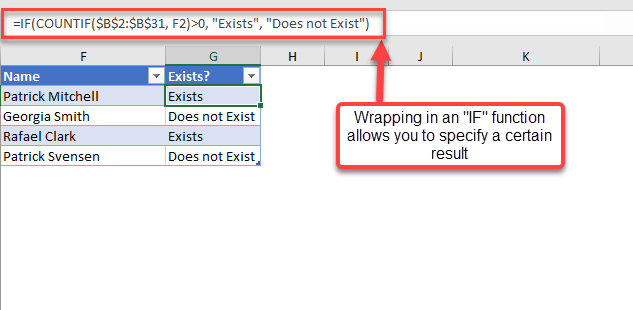## COUNTIF Value Exists in a Range Google Sheets

We use the same formula structure in Google Sheets:

``=IF(COUNTIF(Range, Criteria)>0, “Exists”, “Does Not Exist”)``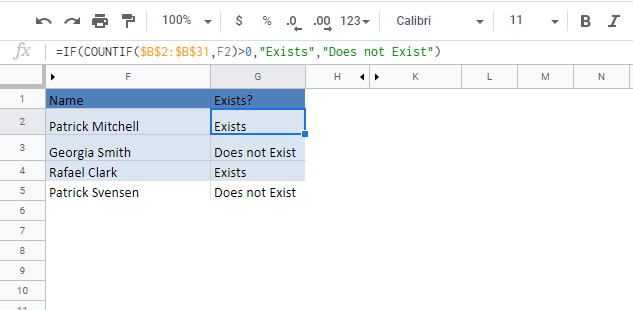### Excel Practice Worksheet

Practice Excel functions and formulas with our 100% free practice worksheets!